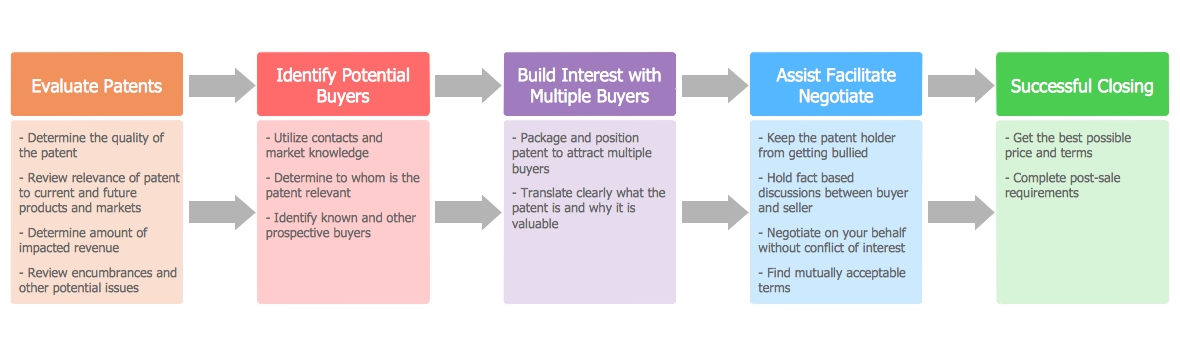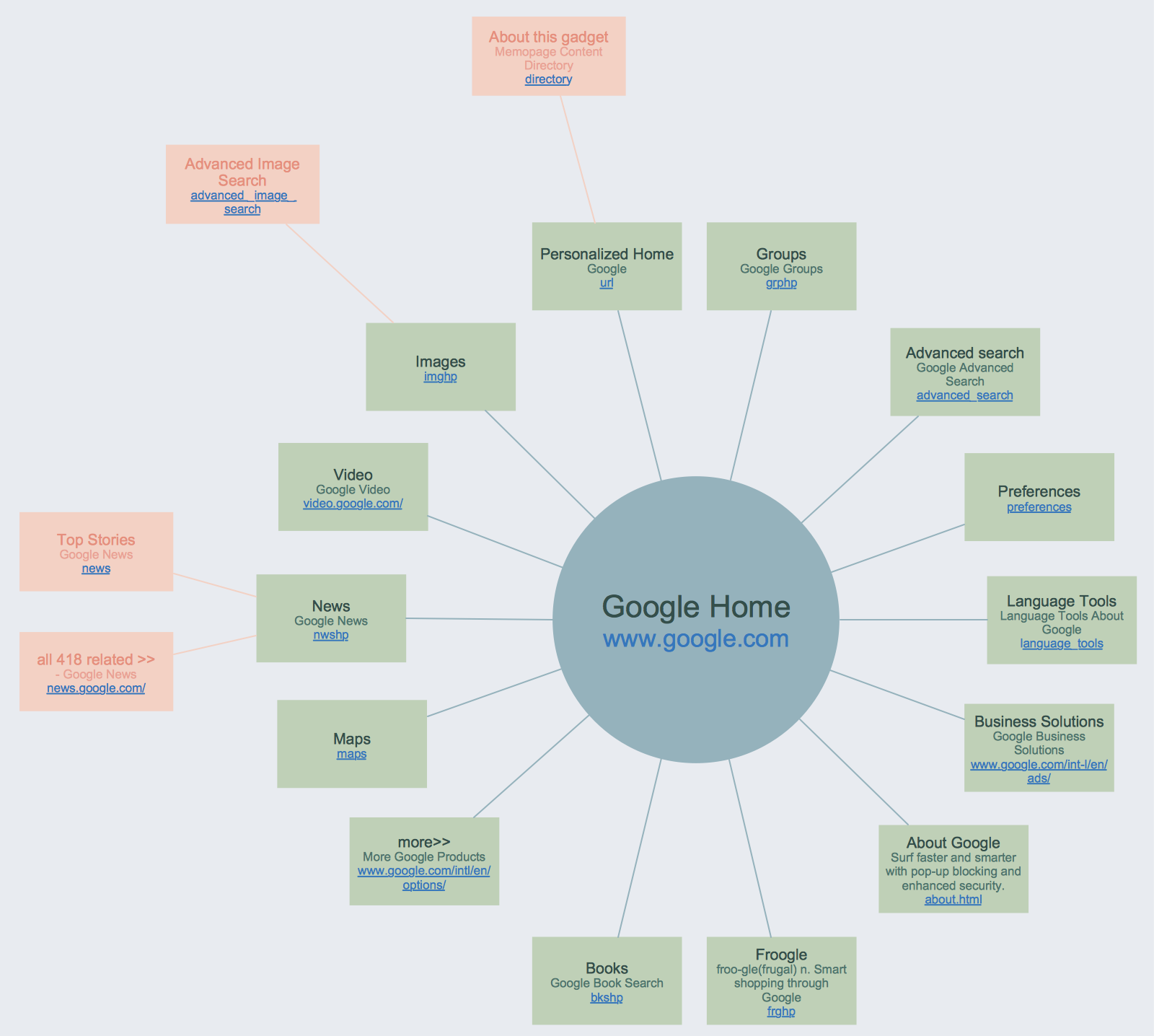This site uses cookies. By continuing to browse the ConceptDraw site you are agreeing to our Use of Site Cookies.
ConceptDraw Samples | Diagrams — Block diagram

# Block Diagrams

Samples of block diagrams are created with ConceptDraw DIAGRAM diagramming and vector drawing software enhanced with Block Diagrams solution from the area "Diagrams" of ConceptDraw Solution Park.

ConceptDraw DIAGRAM provides export of vector graphic multipage documents into multiple file formats: vector graphics (SVG, EMF, EPS), bitmap graphics (PNG, JPEG, GIF, BMP, TIFF), web documents (HTML, PDF), PowerPoint presentations (PPT), Adobe Flash (SWF).

## Tutorials and Solutions:

Video Tutorials: ConceptDraw Solution Park

Solutions: Block Diagrams for ConceptDraw DIAGRAM

## Sample 1: Automotive HVAC System

Block Diagrams sample: Automotive HVAC system.

This example is created using ConceptDraw DIAGRAM diagramming software enhanced with Block Diagrams solution from ConceptDraw Solution Park.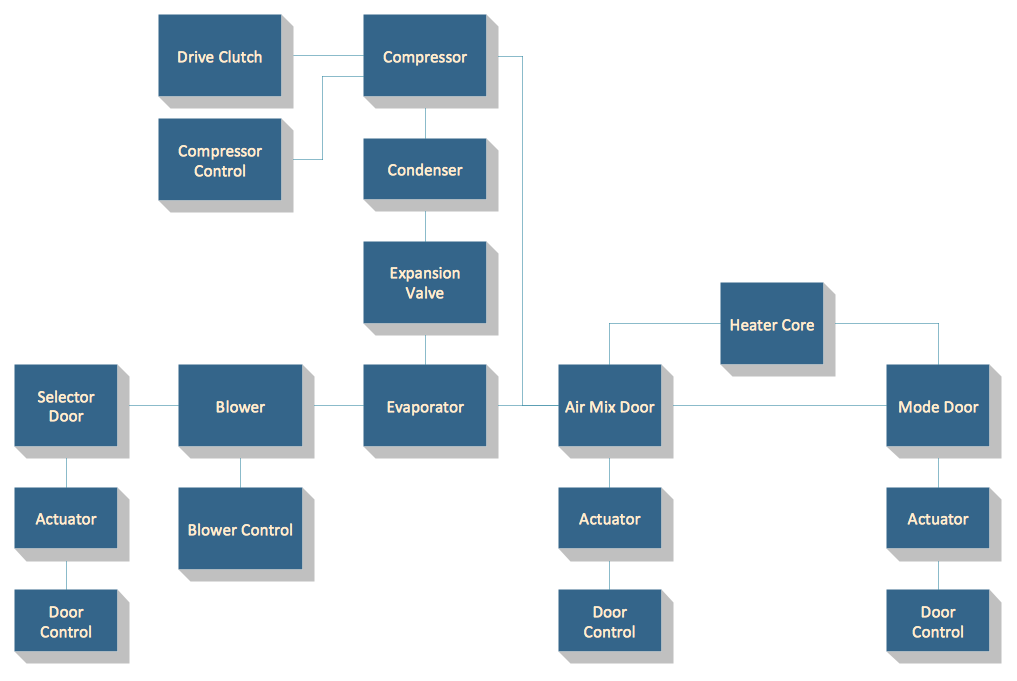## Sample 2: Branding Strategies

Block Diagrams sample: Branding strategies.

This example is created using ConceptDraw DIAGRAM diagramming software enhanced with Block Diagrams solution from ConceptDraw Solution Park.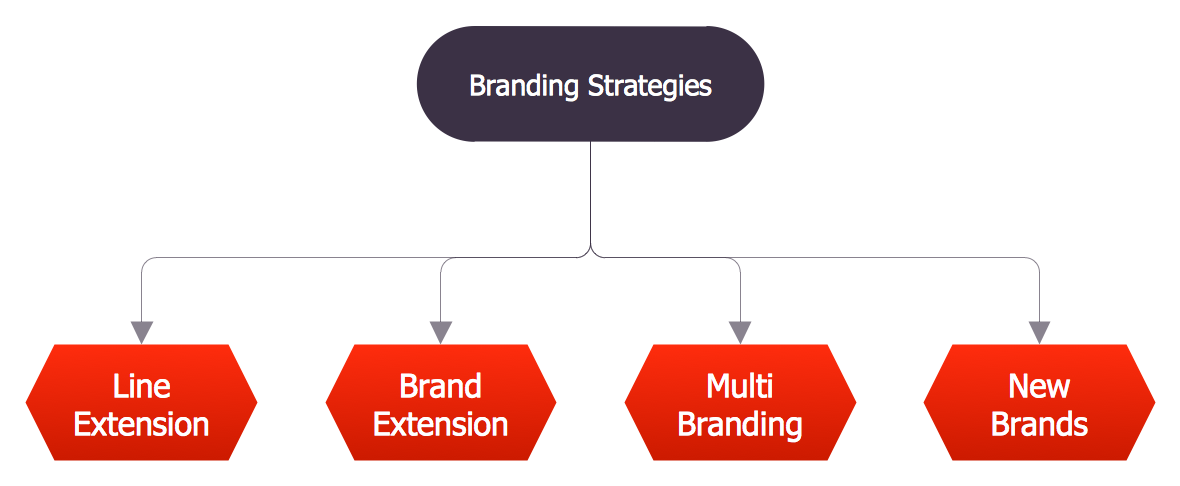## Sample 3: Customer Decision Making

Block Diagrams sample: Customer decision making.

This example is created using ConceptDraw DIAGRAM diagramming software enhanced with Block Diagrams solution from ConceptDraw Solution Park.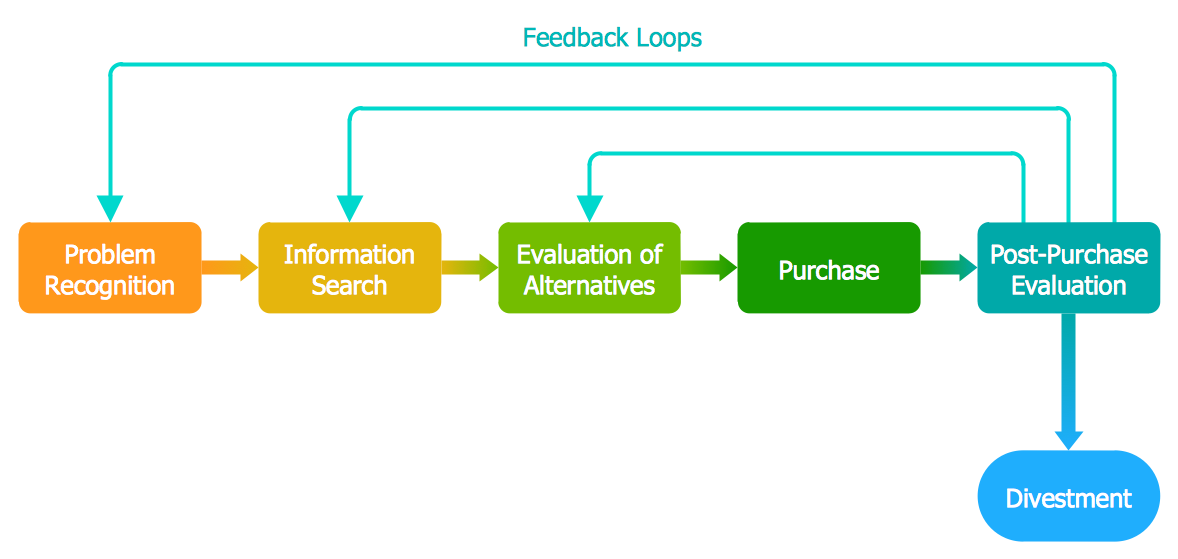## Sample 4: Document Management System Architecture

Block Diagrams sample: Document management system architecture.

This example is created using ConceptDraw DIAGRAM diagramming software enhanced with Block Diagrams solution from ConceptDraw Solution Park.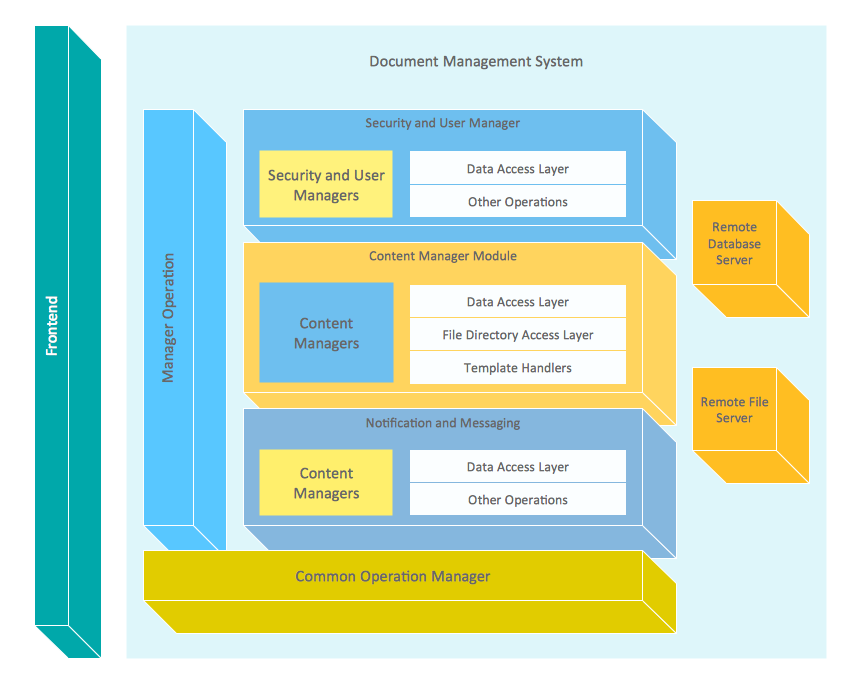## Sample 5: Gap Model of Service Quality

Block Diagrams sample: Gap model of service quality.

This example is created using ConceptDraw DIAGRAM diagramming software enhanced with Block Diagrams solution from ConceptDraw Solution Park.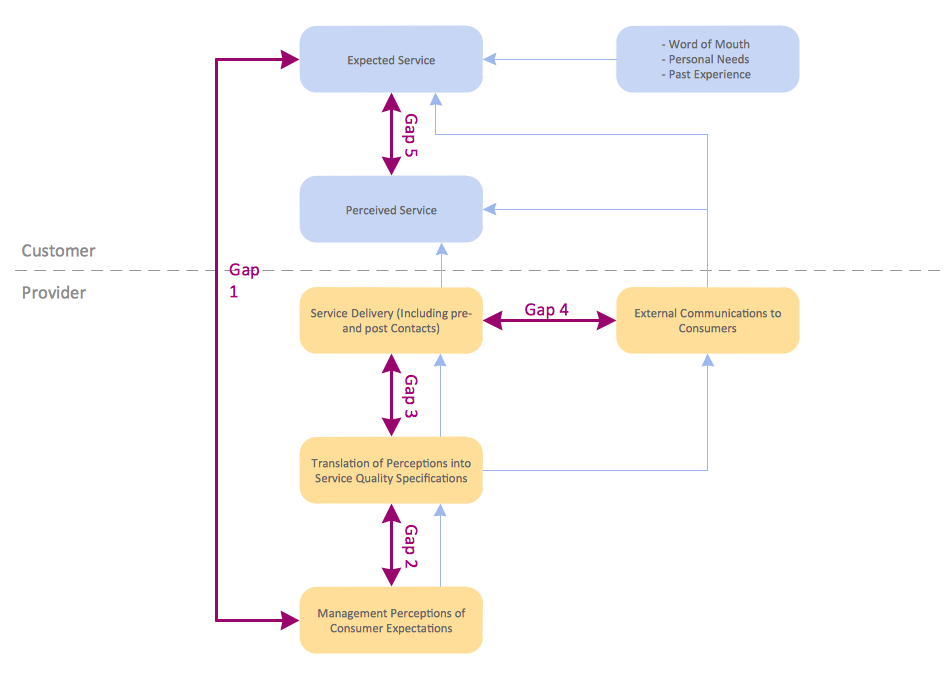## Sample 6: Market Targeting

Block Diagrams sample: Market targeting.

This example is created using ConceptDraw DIAGRAM diagramming software enhanced with Block Diagrams solution from ConceptDraw Solution Park.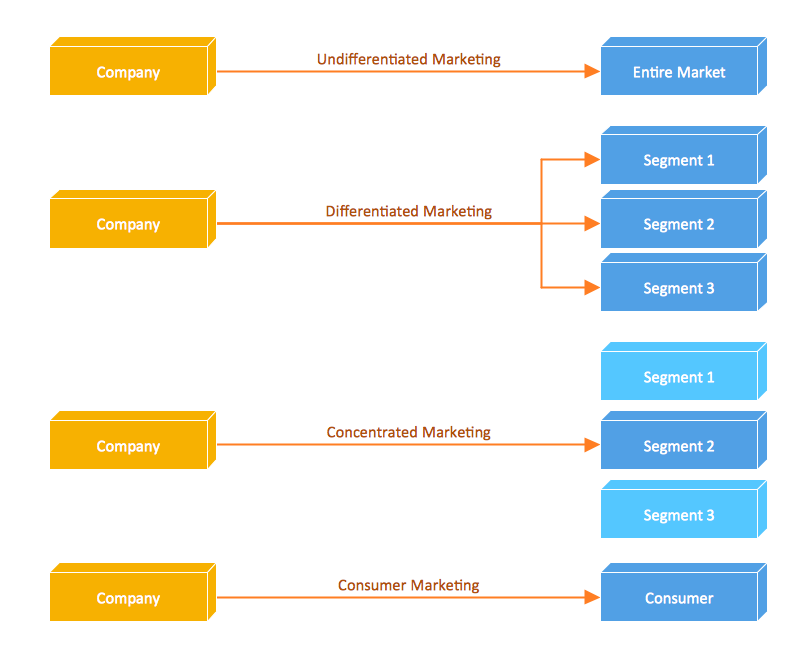## Sample 7: Planning Process

Block Diagrams sample: Planning process.

This example is created using ConceptDraw DIAGRAM diagramming software enhanced with Block Diagrams solution from ConceptDraw Solution Park.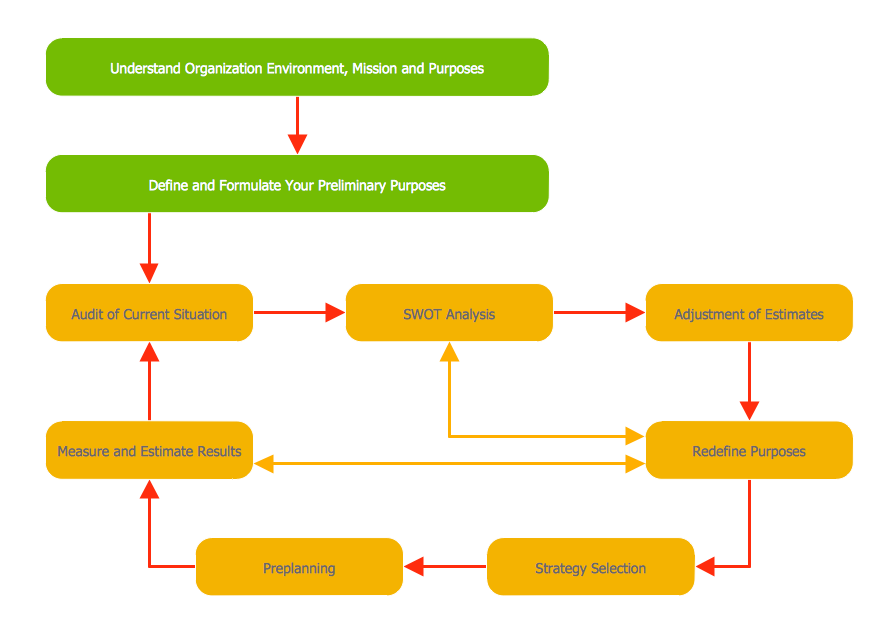## Sample 8: Porter's Five Forces Model

Block Diagrams sample: Porter's five forces model.

This example is created using ConceptDraw DIAGRAM diagramming software enhanced with Block Diagrams solution from ConceptDraw Solution Park.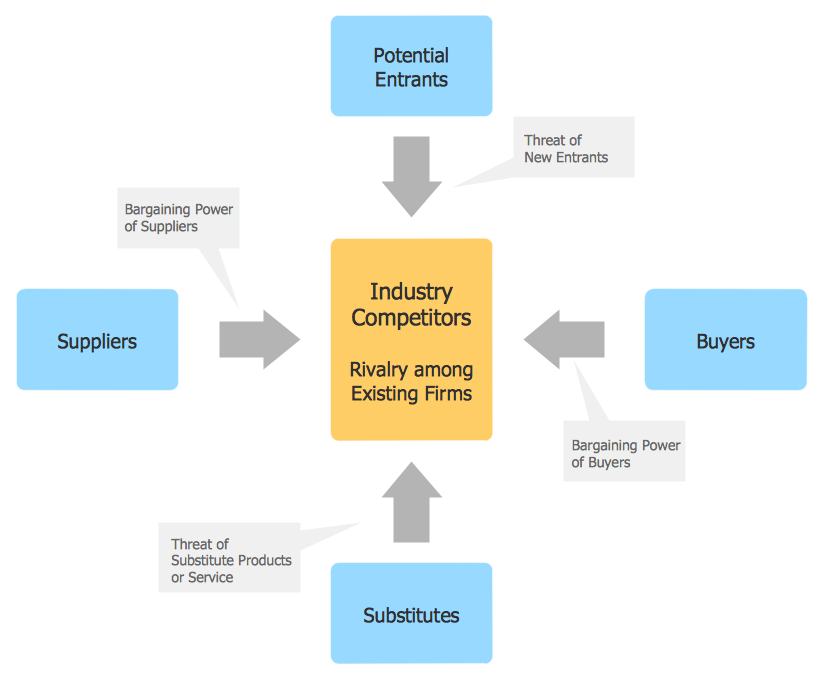## Sample 9: Six Markets Model

Block Diagrams sample: Six markets model.

This example is created using ConceptDraw DIAGRAM diagramming software enhanced with Block Diagrams solution from ConceptDraw Solution Park.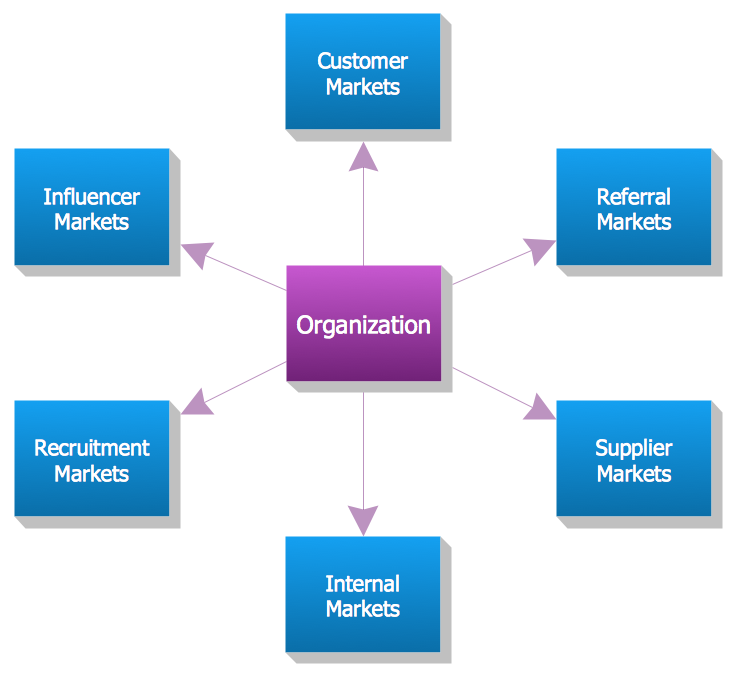## Sample 10: Sources of Customer Satisfaction

Block Diagrams sample: Sources of Customer Satisfaction.

This example is created using ConceptDraw DIAGRAM diagramming software enhanced with Block Diagrams solution from ConceptDraw Solution Park.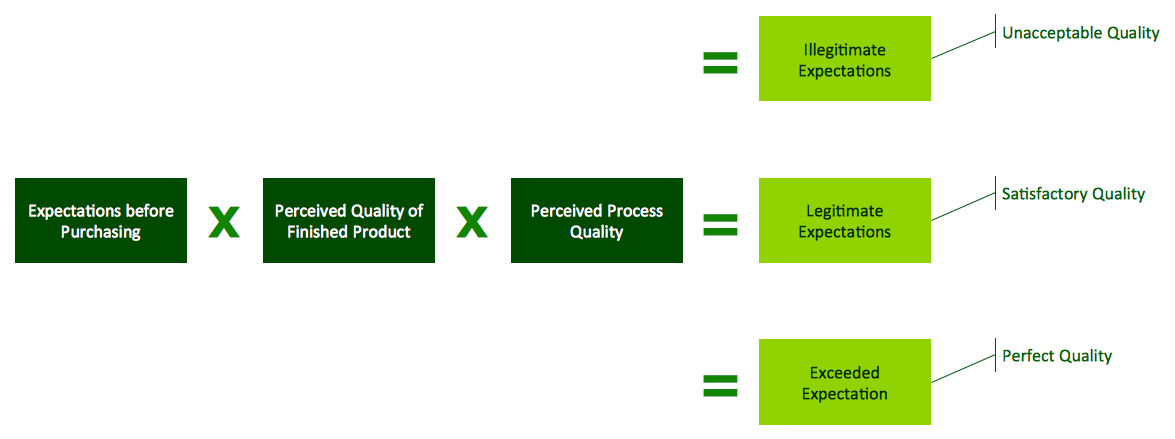## Sample 11: Stages of Promise Issue

Block Diagrams sample: Stages of promise issue.

This example is created using ConceptDraw DIAGRAM diagramming software enhanced with Block Diagrams solution from ConceptDraw Solution Park.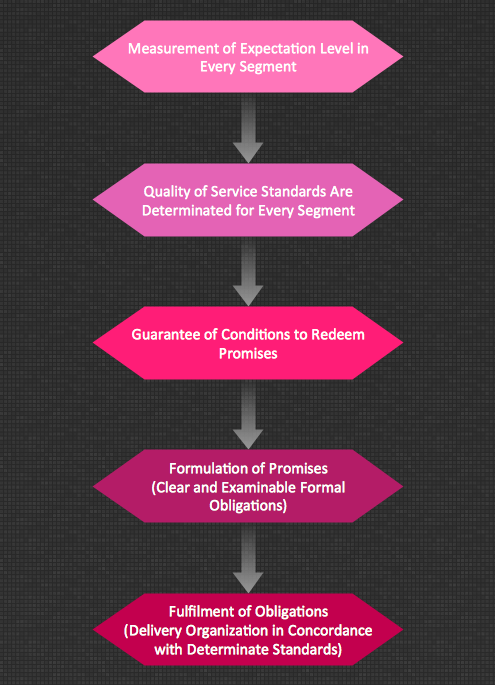## Sample 12: Total Solution Process

Block Diagrams sample: Total solution process.

This example is created using ConceptDraw DIAGRAM diagramming software enhanced with Block Diagrams solution from ConceptDraw Solution Park.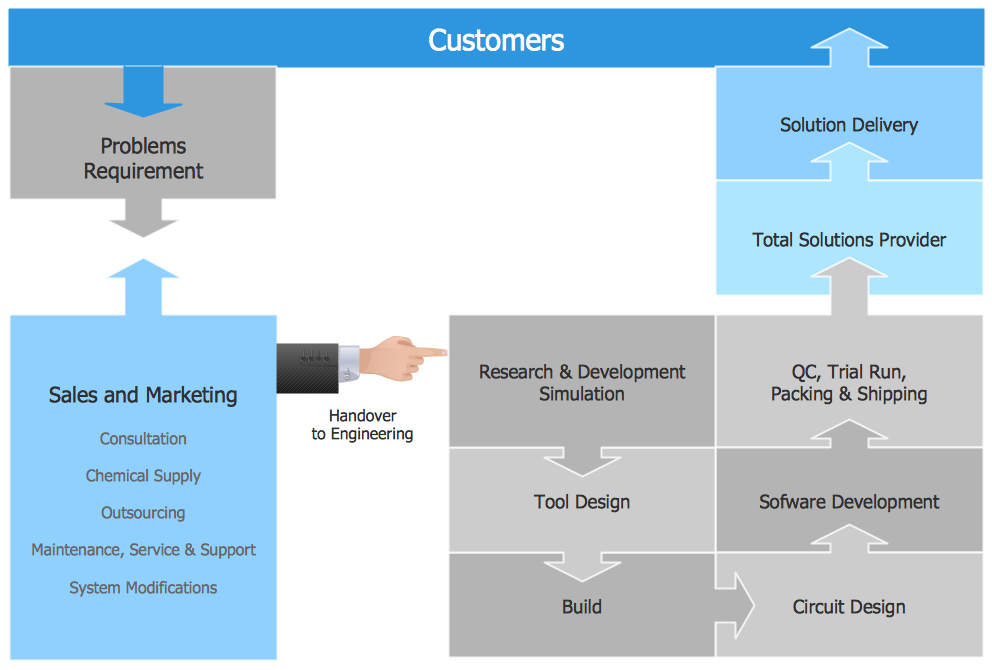## Sample 13: Types of Individual Behavior in Organization

Block Diagrams sample: Types of individual behavior in organization.

This example is created using ConceptDraw DIAGRAM diagramming software enhanced with Block Diagrams solution from ConceptDraw Solution Park.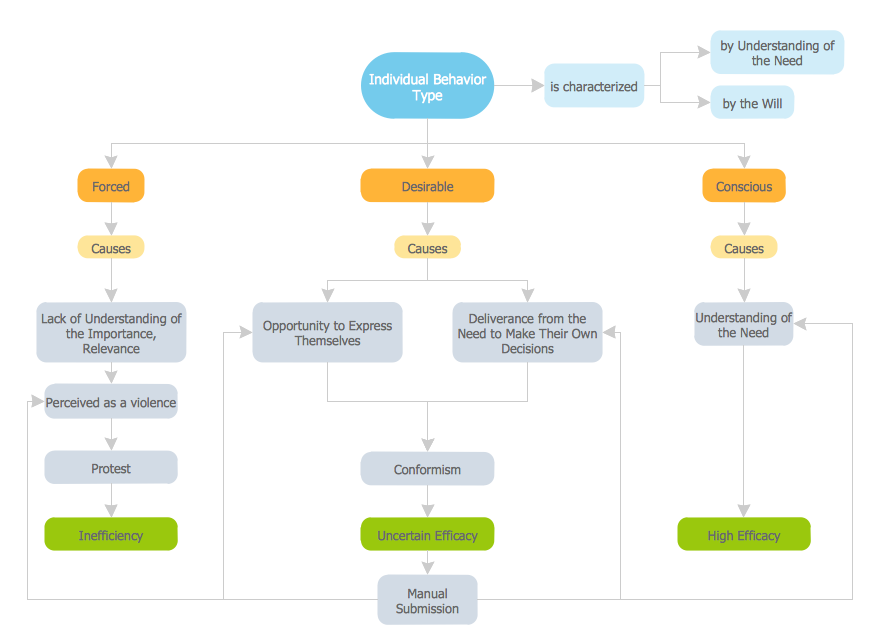## Sample 14: Winning Qualities

Block Diagrams sample: Winning qualities.

This example is created using ConceptDraw DIAGRAM diagramming software enhanced with Block Diagrams solution from ConceptDraw Solution Park.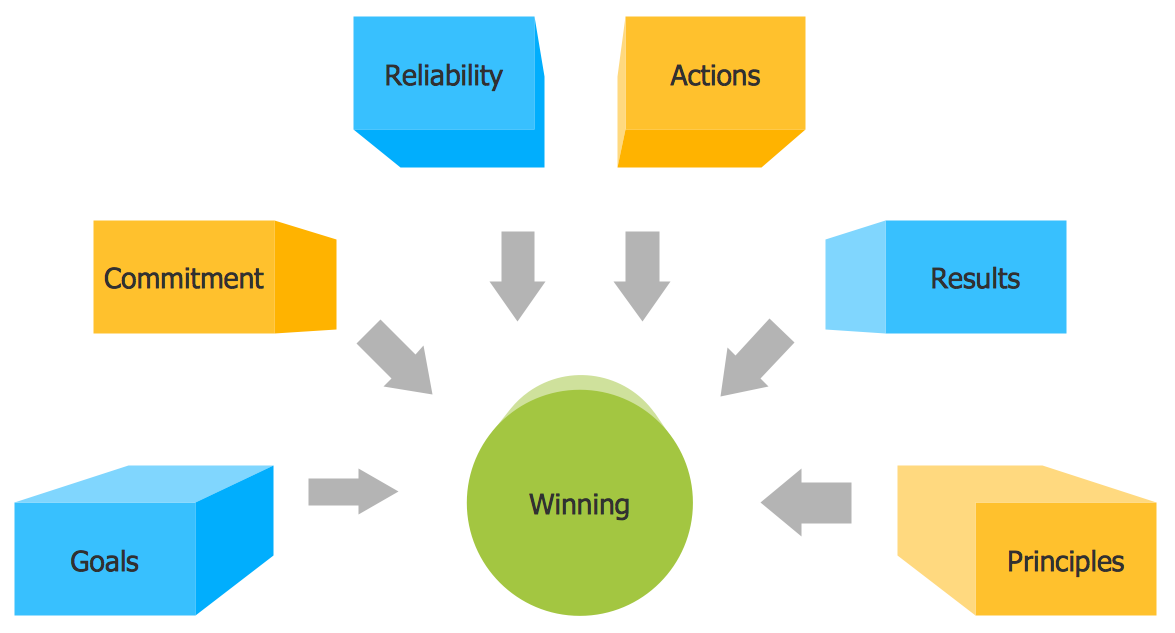## Sample 15: Step Process Chart — Selling Technology Patents Process

Block Diagrams sample: Step process chart — selling technology patents process.

This example is created using ConceptDraw DIAGRAM diagramming software enhanced with Block Diagrams solution from ConceptDraw Solution Park.